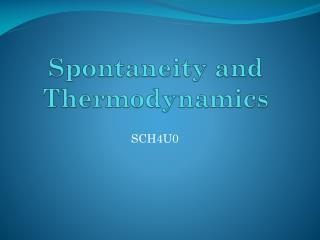Download PresentationSpontaneity and Thermodynamics

# Spontaneity and Thermodynamics

Download Presentation## Spontaneity and Thermodynamics

- - - - - - - - - - - - - - - - - - - - - - - - - - - E N D - - - - - - - - - - - - - - - - - - - - - - - - - - -
##### Presentation Transcript

1. Spontaneity • Recall the combustion of methane • The products contain lower potential energy than the reactants • The reaction is exothermic CH4, 2 O2 ΔH PE CO2, 2 H2O

2. Spontaneity • Since the products have lower potential energy than the reactants, this reaction occurs spontaneously • Meaning that it happens naturally without any human interference • This suggests that; • Exothermic reactions are always spontaneous • Endothermic reactions are always spontaneous • But this is not always the case • Why not?

3. Entropy • There is a second quantity of energy (other than enthalpy) that changes in chemical reactions that affects the spontaneity of a reaction • Entropy (S) • The measure of randomness or disorder • Large entropy implies a large amount of disorder • This means that the atoms are not ordered, and are free to move in any way • Low entropy implies a small amount of disorder • This means that the atoms are highly ordered, and have restricted motion • Every compound has an associated entropy

4. Entropy • Large molecules have low entropy while small molecules have high entropy • Large molecules have several atoms placed in a specific order • Small molecules only have a few atoms placed in a specific order Glucose (C6H12O6) has low entropy Carbon dioxide has high entropy

5. 2nd Law of Thermodynamics • The entropy of the universe is always increasing, or remaining constant • This means that processes in the universe tend to increase in entropy (get more disordered) • The spontaneous direction for a reaction to proceed is the way that increases the entropy of the system • This in turn increases the entropy of the universe

6. The 2nd Law • The 2nd law does not imply that reactions cannot decrease in entropy (get more ordered) • If that was the case, biological molecules (and thus life) could never have formed • Processes that decrease in entropy are only possible if they are accompanied by equal or greater increases in the entropy of the universe • Eg: Humans building a house decreases entropy, but they must break down glucose to do this (increase entropy)

7. Change in Entropy ΔS • ΔS > 0 if; • There are more moles of the products than reactants • Complex molecules are broken into smaller molecules • A substance changes state from a more ordered state to a less ordered state • solid to liquid, solid to gas, or liquid to gas

8. States Low S High S

9. Gibbs Free Energy • There are two types of energy exchanged in a chemical reaction • Enthalpy (H) and entropy (S) • Gibbs Free Energy (G) is the combination of both enthalpy and entropy • Definition: The amount of energy available to do work in chemical system • This means that G is the energy that can be released from a chemical system and used to do work on the surroundings

10. Gibbs Free Energy • The change in Gibbs free energy for a reaction depends on the enthalpy change, entropy change, and the temperature • Spontaneity • This means that to determine whether or not a reaction is spontaneous we must think about all three of these variable

11. Spontaneity Reactants • Exergonic reactions: • Release energy and are therefore spontaneous • ΔG < 0 • Endergonic reactions: • Absorb energy and are therefore non-spontaneous • ΔG > 0 ΔG G Products Products G ΔG Reactants

12. Spontaneity • When is this reaction spontaneous? • Enthalpy is negative – spontaneous • Entropy is positive – spontaneous • At all temperatures this reaction is spontaneous

13. Spontaneity • When is this reaction spontaneous? • Enthalpy is positive – non-spontaneous • Entropy is negative – non-spontaneous • At all temperatures this reaction is non-spontaneous

14. Spontaneity • When is this process spontaneous? • Enthalpy is positive – non-spontaneous • Entropy is positive –spontaneous • If temp. is high, this reaction is spontaneous • If temp. is low, this reaction is non-spontaneous

15. Spontaneity • When is this reaction spontaneous? • Enthalpy is negative – spontaneous • Entropy is negative – non-spontaneous • If temp. is low, this reaction is spontaneous • If temp. is high, this reaction is non-spontaneous